# Test: Differentiation Of Exponential Functions

## 10 Questions MCQ Test Mathematics (Maths) Class 12 | Test: Differentiation Of Exponential Functions

Description
This mock test of Test: Differentiation Of Exponential Functions for JEE helps you for every JEE entrance exam. This contains 10 Multiple Choice Questions for JEE Test: Differentiation Of Exponential Functions (mcq) to study with solutions a complete question bank. The solved questions answers in this Test: Differentiation Of Exponential Functions quiz give you a good mix of easy questions and tough questions. JEE students definitely take this Test: Differentiation Of Exponential Functions exercise for a better result in the exam. You can find other Test: Differentiation Of Exponential Functions extra questions, long questions & short questions for JEE on EduRev as well by searching above.
QUESTION: 1

Solution:
QUESTION: 2

### Differentiate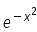with respect to x.

Solution:

y = e^(-x)2.................(1)
Put u = (-x)2
du/dx = -2x dx
Differentiating eq(1) y = eu
dy/du = e^u
⇒ dy/dx = (dy/du) * (du/dx)
= (eu) * (-2x)
⇒ - 2xe(-x2)

QUESTION: 3

### The derivatve of f(x) =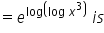Solution:

f(x) =   elog(logx3)
As log and e are inverse operations, therefore
f(x)= logx3
So derivative will be                         {Derivative of log x is 1/x and then applying chain rule}

f’(x)=1/x3. 3x= 3/x

QUESTION: 4

The sum of Which series is denoted by e.

Solution:
QUESTION: 5

Derivatve of f(x)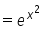is given by

Solution:

yThe derivative of y = ef(x)is dy/dx = f'(x)ef(x)
In this case, f(x) = x2 , and the derivative of x2 = 2x
Therefore, f'(x)= 2x,
dy/dx = 2x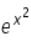QUESTION: 6

The differential equation of y =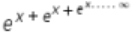Solution:
QUESTION: 7

If f(x) = e2x-5, then f'(x) is​

Solution:
QUESTION: 8

Whta is the derivatve of y = log5 (x)

Solution:

y = log5 x = ln x/ln 5 → change of base
= ln x/ln 5
dy/dx = 1/ln5⋅1/x → 1/ln5 is a constant, so we don't change it
= 1/(x ln 5)

QUESTION: 9

For what values of x is elogx = x

Solution:
QUESTION: 10

If f(x) = ex, then the value of f'(-3) is

Solution:

As, f (x) = ex
Similarly, f (-3) = e-3